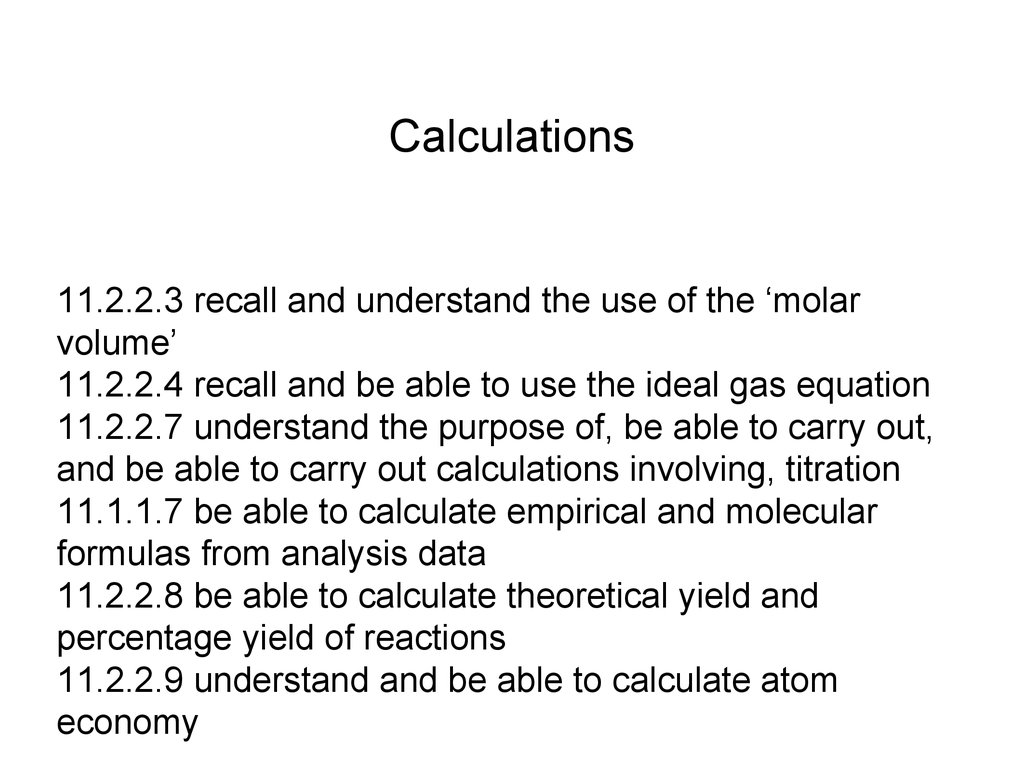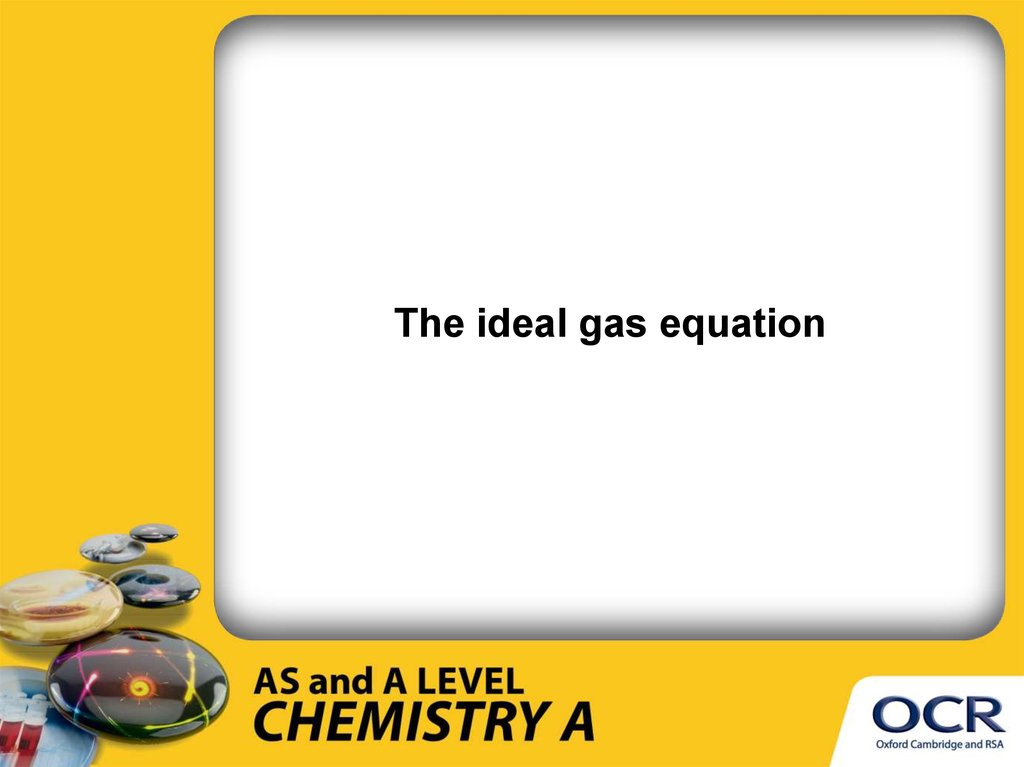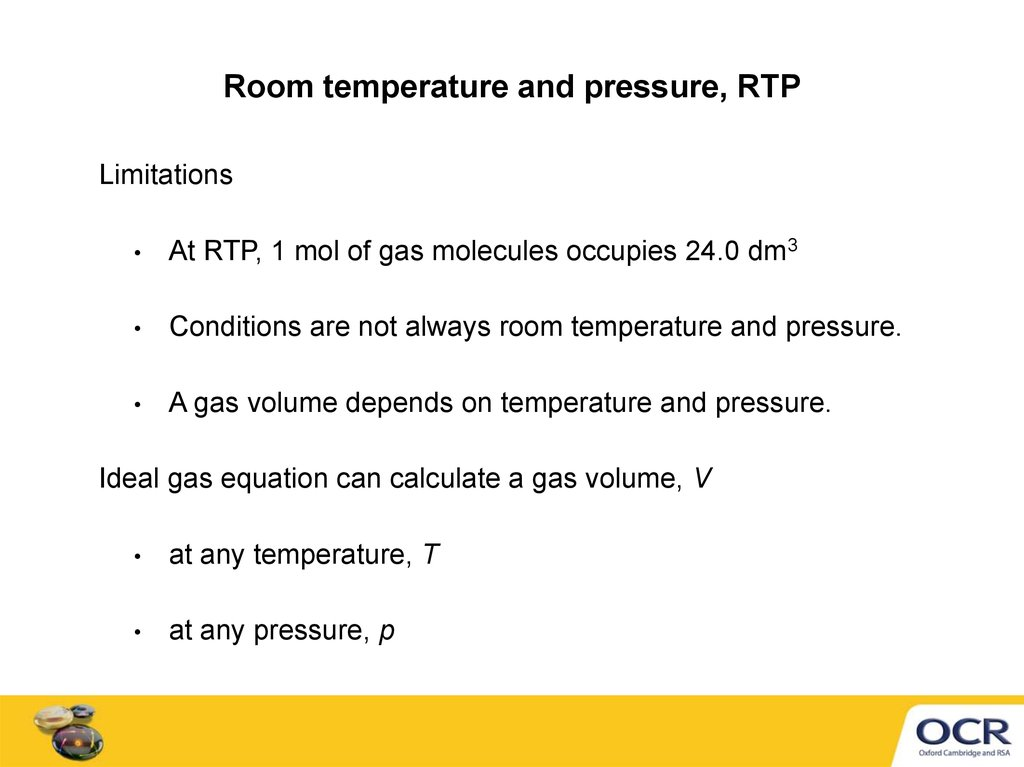# The ideal gas equation

## 1. 11.2.2.3 recall and understand the use of the ‘molar volume’ 11.2.2.4 recall and be able to use the ideal gas equation 11.2.2.7

Calculations
11.2.2.3 recall and understand the use of the ‘molar
volume’
11.2.2.4 recall and be able to use the ideal gas equation
11.2.2.7 understand the purpose of, be able to carry out,
and be able to carry out calculations involving, titration
11.1.1.7 be able to calculate empirical and molecular
formulas from analysis data
11.2.2.8 be able to calculate theoretical yield and
percentage yield of reactions
11.2.2.9 understand and be able to calculate atom
economy

## 2.

The ideal gas equation

## 3.

Room temperature and pressure, RTP
Limitations
At RTP, 1 mol of gas molecules occupies 24.0 dm3
Conditions are not always room temperature and pressure.
A gas volume depends on temperature and pressure.
Ideal gas equation can calculate a gas volume, V
at any temperature, T
at any pressure, p

## 4.

The ideal gas equation
pV = nRT

## 5. Converting units for pV = nRT

Before using pV = nRT, convert units to m3, K and Pa
Examples
cm3 to m3
× 10−6
220 cm3 = 220 × 10−6 cm3
4.0 dm3
= 4.0 × 10−3 m3
dm3 to m3
× 10−3
°C to K
+ 273
48 °C = 48 + 273 = 321 K
kPa to Pa
×
100 kPa
103
= 100 × 103 Pa

## 7.

Calculating a relative molecular mass

## 9.

AN INTRODUCTION TO
ATOM ECONOMY
KNOCKHARDY PUBLISHING

## 10.

ATOM ECONOMY
In most reactions you only want to make one of the resulting products
Atom economy is a measure of how much of the products are useful
A high atom economy means that there is less waste

## 11.

ATOM ECONOMY
In most reactions you only want to make one of the resulting products
Atom economy is a measure of how much of the products are useful
A high atom economy means that there is less waste
ATOM ECONOMY
MOLECULAR MASS OF DESIRED PRODUCT
x
100

## 12.

Example
1
WORKED CALCULATIONS
Calculate the atom economy for the formation of 1,2-dichloroethane, C2H4Cl2

## 13.

Example
1
WORKED CALCULATIONS
Calculate the atom economy for the formation of 1,2-dichloroethane, C2H4Cl2
Equation C2H4
Mr
+
28
atom economy
——>
Cl2
71
=
C2H4Cl2
99
molecular mass of C2H4Cl2
= 99
x
100
=
100%
99
An ATOM ECONOMY of 100% is
x 100

## 14.

Example
2
WORKED CALCULATIONS
Calculate the atom economy for the formation of nitrobenzene, C6H5NO2

## 15.

Example
2
WORKED CALCULATIONS
Calculate the atom economy for the formation of nitrobenzene, C6H5NO2
HNO3 ——>
Equation C6H6 +
Mr
78
atom economy
C6H5NO2
63
=
+
123
H 2O
18
molecular mass of C6H5NO2
=
123
x 100
=
87.2%
123 + 18
An ATOM ECONOMY of 100% is not possible
with a SUBSTITUTION REACTION
x 100

## 16.

Example
3
WORKED CALCULATIONS
Calculate the atom economy for the preparation of ammonia from the thermal
decomposition of ammonium sulphate.

## 17.

Example
3
WORKED CALCULATIONS
Calculate the atom economy for the preparation of ammonia from the thermal
decomposition of ammonium sulphate.
Equation (NH4)2SO4
Mr
——>
132
98
atom economy
=
H2SO4
+
2NH3
17
2 x molecular mass of NH3
x 100
=
2 x 17
= 25.8%
98 + (2 x 17)
In industry a low ATOM ECONOMY isn’t necessarily
that bad if you can use some of the other products. If
this reaction was used industrially, which it isn’t, the
sulphuric acid would be a very useful by-product.

## 18.

CALCULATIONS
Calculate the atom economy of the following reactions (the required product is
shown in red)
• CH3COCl + C2H5NH2
——>
CH3CONHC2H5 + HCl
• C2H5Cl + NaOH
——>
C2H5OH + NaCl
• C2H5Cl + NaOH
——>
C 2H 4 + H 2O
+
NaCl

## 19.

CALCULATIONS
Calculate the atom economy of the following reactions (the required product is
shown in red)
• CH3COCl + C2H5NH2
——>
CH3CONHC2H5 + HCl
• C2H5Cl + NaOH
——>
C2H5OH + NaCl
• C2H5Cl + NaOH
——>
C 2H 4 + H 2O
+
NaCl
70.2
%

## 20.

CALCULATIONS
Calculate the atom economy of the following reactions (the required product is
shown in red)
• CH3COCl + C2H5NH2
——>
CH3CONHC2H5 + HCl
• C2H5Cl + NaOH
——>
C2H5OH + NaCl
• C2H5Cl + NaOH
——>
C 2H 4 + H 2O
+
NaCl
70.2
%
55.8
%
33.9
%

## 21.

OVERVIEW
• addition reactions will have 100% atom economy
• substitution reactions will have less than 100% atom economy
• high atom economy = fewer waste materials
= GREENER and MORE ECONOMICAL
The percentage yield of a reaction must also be taken into consideration.
• some reactions may have a high yield but a low atom economy
• some reactions may have a high atom economy but a low yield
Reactions involving equilibria must also be considered

## 22.

Percentage yield
Perform calculations to determine the percentage
yield of a reaction

## 23.

In a chemical reaction which is totally efficient
all the REACTANTS are converted into
products.
This will give 100% yield.
Most reactions, particularly organic reactions
give low yields.
Possible reasons:
Impure reactants.
Product is lost during purification.
Side reactions.
Equilibrium reaction means that a reaction is
never completed.

## 24. Definitions

Know that:
The theoretical yield is the maximum mass of products
which would be obtained from the balanced equation.
The actual yield is the mass of products obtained.
The percentage yield = Actual yield
Theoretical yield
Limiting reactant is the substance present in lowest quantity
which determines the actual yield.
Excess – more than the mass determined by the balanced
equation is used to maximise product obtained.
x 100%

## 25.

Calculating Percentage (%) Yield
2.3g of sodium reacts with an excess
of chlorine to produce 4.0g of sodium chloride.
What is the percentage yield?
2Na(s)
+
Cl2(g)
2NaCl(s)
(Ar reactants: Na=23 Cl=35.5
2.3g Na = 2.3 mol Na
23
Mr product: NaCl= 58.5)
= 0.1 mol Na
Theoretically 0.1 mol Na should yield 0.1 mol NaCl
Theoretical yield of NaCl = 58.5 x 0.1 = 5.85g
% Yield = Actual yield x 100%
Theoretical yield
% Yield = 4.0g x 100% = 68%
5.85g

## 26.

Calculating Percentage (%) Yield
If 1.2g of magnesium reacts with an excess
of oxygen to produce 0.8g of magnesium
oxide…
What is the percentage yield?
2Mg(s) +
O2(g)
2MgO(s)
(Ar reactants: Mg=24 O=16
Mr product: MgO= 40)
1.2g Mg = 1.2 mol Mg = 0.05 mol Mg
24
Theoretically 0.05 mol Mg should yield 0.05 mol MgO
Theoretical yield of MgO = 40 x 0.05 = 2g
% Yield = Actual yield x 100%
Theoretical yield
% Yield = 0.8g x 100% = 40%
2g

## 27.

Calculating Percentage (%) Yield
If 2g of calcium carbonate reacts with an excess of hydrochloric
acid to produce 1.11 g of calcium chloride….
What is the percentage yield?
2HCl(aq)+ CaCO3(s) H2O(l) + CO2(g) + CaCl2(s)
(Mr values are: CaCO3 = 100 CaCl2 = 111)
2g CaCO3 = 2 mol CaCO3 = 0.02 mol CaCO3
100
Theoretically 0.02 mol CaCO3 should yield 0.02 mol CaCl2
Theoretical Yield of CaCl2 = 111 x 0.02 = 2.22g
% Yield = Actual yield x 100%
Theoretical yield
% Yield = 1.11 x 100 = 50%
2.22